## Things are not as straightforward as they seem

Consider the blow-up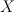$X$ of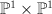$\PP^1 \times \PP^1$ with center a complete intersection of type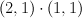$(2,1)\cdot(1,1)$.  Since the complete intersection consists of three points,$X$ is a del Pezzo surface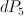$dP_5$.  It is tempting to compute its regularized period sequence as follows.

Warning: this calculation is wrong. I explain below where the error is and how to fix it.  We express$X$ as a complete intersection in a toric variety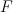$F$ as follows.  Let$F$ have weight data: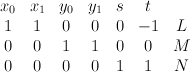$\begin{array}{ccccccc} x_0 & x_1 & y_0 & y_1 & s & t & \\ 1 & 1 & 0 & 0 & 0 & -1 & L \\ 0 & 0 & 1 & 1 & 0 & 0 & M \\ 0 & 0 & 0 & 0 & 1 & 1 & N \end{array}$
Now consider the equation: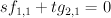$s f_{1,1} + t g_{2,1} = 0$
where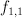$f_{1,1}$ and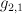$g_{2,1}$ are polynomials in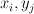$x_i, y_j$ of bidegrees (respectively) (1,1) and (2,1).  The variety$X$ defined by this equation is cut out by a section of the line bundle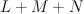$L+M+N$; by projecting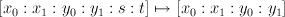$[x_0:x_1:y_0:y_1:s:t] \mapsto [x_0:x_1:y_0:y_1]$ we see that$X$ is, as desired, the blow-up of$\PP^1 \times \PP^1$ in a complete intersection of type$(2,1)\cdot(1,1)$.  We have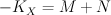$-K_X = M+N$ and: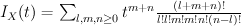$I_X(t) = \sum_{l,m,n \geq 0} t^{m+n} {(l+m+n)! \over l!l!m!m!n!(n-l)!}$
Regularizing gives the period sequence: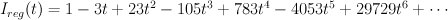$I_{reg}(t) = 1-3 t+23 t^2-105 t^3+783 t^4-4053 t^5+29729 t^6+\cdots$

This is not correct: we know the regularized period sequences for del Pezzo surfaces, and in the case of$dP_5$ we get: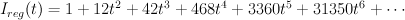$I_{reg}(t) =1+12 t^2+42 t^3+468 t^4+3360 t^5+31350 t^6 + \cdots$
So what went wrong?

The construction of$X$ given above is correct.  It is the second half of the calculation which is flawed.  The key point is that, even though$X$ is Fano and is cut out of the ambient space$F$ by a section of an ample line bundle,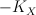$-K_X$ is not the restriction of an ample line bundle on$F$ but rather is only the restriction of a semi-positive line bundle on$F$Thus the mirror map is non-trivial. To see this we need to consider not Golyshev’s I-function: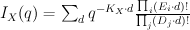$I_X(q) = \sum_{d} q^{-K_X\cdot d} {\prod_{i} (E_i\cdot d)! \over \prod_j (D_j \cdot d)!}$
but rather the full Givental I-function: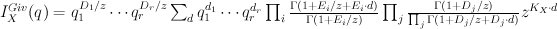$I^{Giv}_X(q) = q_1^{D_1/z}\cdots q_r^{D_r/z} \sum_{d} q_1^{d_1}\cdots q_r^{d_r} \prod_{i} {\Gamma(1+E_i/z+E_i\cdot d) \over \Gamma(1+E_i/z)} \prod_j {\Gamma(1+D_j/z) \over \prod_j \Gamma(1+D_j/z+D_j \cdot d)} z^{K_X \cdot d}$
Here$X$ is cut out of the toric variety with toric divisors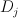$D_j$ by a section of the direct sum of line bundles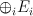$\oplus_i E_i$.  Golyshev’s I-function is obtained from Givental’s I-function by taking the term in cohomological degree zero and setting: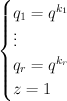$\begin{cases} q_1 = q^{k_1} \\ \vdots \\ q_r = q^{k_r} \\ z = 1 \end{cases}$
where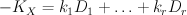$-K_X = k_1 D_1 + \ldots + k_r D_r$.  Note that Givental’s I-function is homogeneous of degree zero if we set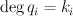$\deg q_i = k_i$,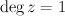$\deg z = 1$, and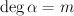$\deg \alpha = m$ whenever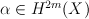$\alpha \in H^{2m}(X)$.

In the situation at hand (i.e.$X$ is a semipositive complete intersection in a toric variety) we have, for grading reasons: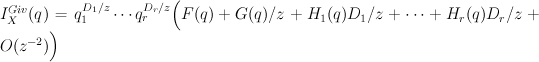$I^{Giv}_X(q) = q_1^{D_1/z}\cdots q_r^{D_r/z} \Big(F(q) + G(q)/z + H_1(q) D_1/z + \cdots + H_r(q) D_r/z + O(z^{-2}) \Big)$
where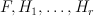$F, H_1,\ldots,H_r$ are degree-zero power series in the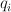$q_i$ and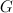$G$ is a degree-1 power series in the$q_i$.  Furthermore Givental’s mirror theorem states that: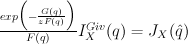${exp\Big(-{G(q) \over z F(q)}\Big) \over F(q)} I^{Giv}_X(q) = J_X(\hat{q})$
where: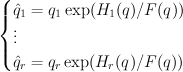$\begin{cases} \hat{q}_1 = q_1 \exp(H_1(q)/F(q)) \\ \vdots \\ \hat{q}_r = q_r \exp(H_r(q)/F(q)) \end{cases}$
This change of variables is called the mirror map.  The regularized quantum period sequence that we seek is obtained from the cohomological-degree-zero  component of the J-function by setting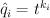$\hat{q}_i = t^{k_i}$,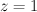$z=1$, and doing the trick with factorials: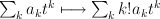$\sum_k a_k t^k \longmapsto \sum_k k! a_k t^k$.

Applying this discussion in our case (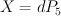$X = dP_5$ realized as above) yields: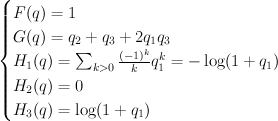$\begin{cases} F(q) = 1 \\ G(q) = q_2+q_3+2q_1 q_3 \\ H_1(q) = \sum_{k>0} {(-1)^{k} \over k} q_1^k = {-\log(1+q_1)} \\ H_2(q) = 0 \\ H_3(q) = \log(1+q_1) \end{cases}$
and hence: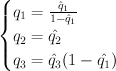$\begin{cases} q_1 = {\hat{q}_1 \over 1 - \hat{q}_1} \\ q_2 = \hat{q_2} \\ q_3 = \hat{q}_3 (1-\hat{q_1}) \end{cases}$
Thus the cohomological-degree-zero part of the J-function is: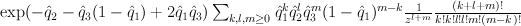$\exp(-\hat{q}_2-\hat{q}_3(1-\hat{q}_1)+2\hat{q}_1 \hat{q}_3) \sum_{k,l,m\geq 0} \hat{q}_1^k \hat{q}_2^l \hat{q}_3^m(1-\hat{q}_1)^{m-k} {1 \over z^{l+m}} {(k+l+m)! \over k!k!l!l!m!(m-k)!}$
and setting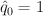$\hat{q}_0 = 1$,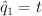$\hat{q}_1 = t$,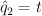$\hat{q}_2 = t$,$z=1$ yields: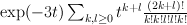$\exp(-3t) \sum_{k,l\geq 0} t^{k+l} {(2k+l)! \over k!k!l!l!k!}$
Regularizing this gives:$I_{reg}(t) =1+12 t^2+42 t^3+468 t^4+3360 t^5+31350 t^6 + \cdots$
which agrees with our previous calculation.

1.Sergey says: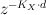$z^{-K_X \cdot d}$ should be$z^{K_X \cdot d}$

Tom, could you please explain if the equivariant redundancy is necessary for the proper mirror map or can be ommited?

In particular, could you please write what is$q_i$ in the definition of Givental’s I-function.
There is a redundancy of these parameters: divisors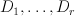$D_1,\dots,D_r$ form a base for space of T-equivariant divisor classes,
but are linearly dependant in the non-equivariant Picard group:$Formula does not parse: Pic(F) = Pic^T(F)/Hom(T,\C^*)$.

Is d any class or a class of effective curve on$F$ and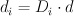$d_i = D_i \cdot d$?

Also, variety F has an action of torus T, but complete intersection X already doesn’t admit such action.
So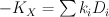$-K_X = \sum k_i D_i$ as an equation in $Pic(X)$ doesn’t define values of k_i,
should we abandon equivariant parameters in any way and restrict ourselves to the non-equivariant only (in the example this is done indeed)?

2.Tom says:

@Sergey: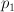$p_1$ is the class Poincare-dual to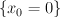$\{x_0=0\}$,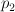$p_2$ is the class Poincare-dual to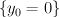$\{y_0=0\}$, and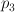$p_3$ is the class Poincare-dual to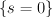$\{s=0\}$. (Thus they correspond to the standard basis when I look at the weight data defining the toric variety$F$.)$q_i$ is dual to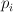$p_i$.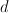$d$ is an effective class and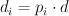$d_i = p_i \cdot d$. As I see it, we do not need to consider the equivariant theory at all.